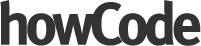1
Francis# Simple Neural Networks explained with Javascript1# Simple Neural Networks explained with Javascript

We hear a lot about Machine Learning and Artificial Intelligence these days, so I thought it would be cool to look at a really simple example using Javascript.

We're going to use the Brain.js library to create our neural network, it takes care of the heavy lifting for us.

#### What is a neural network?

A neural network is an algorithmic construct that's loosely modelled after the human brain. They're built using layers. There's an input layer, an output layer and one or more layers in between to do the processing.

The layers contain the 'neurons'. As the data moves across the layers its value is multiplied by certain weights which determine how much impact each neuron has on the output.

We 'train' our network by adjusting these weights.

We provide our inputs to the input layer, the data from the input layer then travels through the neural network across all of its layers to the output layer, where it gives us the probability of each output being the correct answer.

#### What will our neural network do?

Our network will approximate the output of the exclusive-or operation (XOR). We'll provide our network with two inputs and the network will predict whether the output should be a 1.

#### What's XOR?

XOR is a binary operation that takes two inputs and produces one output. If the inputs are the same, it'll output a 0 and if the inputs are different it'll output a 1.

Below is the truth table for XOR.

```A | B | Output
--------------
0 | 0 |   0
0 | 1 |   1
1 | 0 |   1
1 | 1 |   0
```

#### The code

```const brain = require('brain.js')

var net = new brain.NeuralNetwork()

var training_data = [
{input: [0, 0], output: },
{input: [0, 1], output: },
{input: [1, 0], output: },
{input: [1, 1], output: }
]

net.train(training_data)

console.log(net.run([0, 0]))
console.log(net.run([1, 0]))
```

#### What's going on?

1. The first thing we do is include the Brain.js library.
2. Next, we create a new Neural Network with `new brain.NeuralNetwork()`
3. Then we define our training data, this is an array of objects. Each object has an input and an output property. The input property gives the network 2 inputs and the output property tells the network what the output should be for those inputs.
4. Next, we train our network.
5. Finally, we run our network by calling `net.run()`

When we run our code we get the following outputs:

```Input: [0, 0] Output: [ 0.05875255912542343 ]
Input: [1, 0] Output: [ 0.9338177442550659 ]
```

You can see our network is working correctly. When our inputs are 0,0 the output is 0.058 which means the probability the output is a 1 is almost 0, which is correct because the correct answer is 0.

And when our inputs are 1,0 the output probability is 93% which means the network is almost certain that the correct answer is 1.

That's a simple look at neural networks! Hopefully, you learned a lot!

• Javascript
Francis# 1 Replies0
SiddharthBoseTypo: We here a lot ....0Typo: We here a lot ....

SiddharthBose